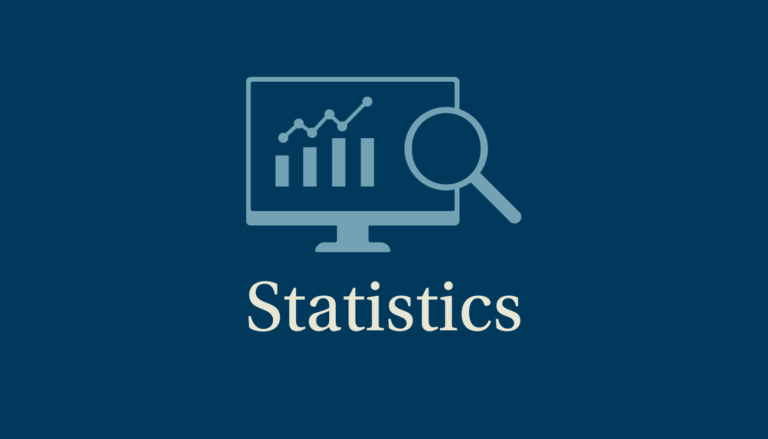# Statistics

## Using and understanding data is becoming increasingly important in many areas of study and employment. Understanding of statistics is a crucial skill, and this specification aims to give students the tools needed to help them prepare for higher education and the workplace.A level Statistics might appeal to the student seeking to pursue the study of a numerate post-16 subject but not wishing to study pure mathematics. It sits well with subjects such as A level Biology, Psychology, Geography, Business Studies and Economics.

The emphasis is on using and applying statistics; appropriate interpretation of contexts and the outcomes of statistical procedures will be required. Content will focus on using distributions to make predictions and completing hypothesis tests to test the validity of assertions made about data in a variety of practical contexts.

Students will sit three papers at the end of their second year, each lasting for two hours.

As part of our commitment to ensuring students enjoy the challenges that studying mathematics brings, we arrange a number of activities throughout the year to enrich their experience.

These include opportunities to apply their knowledge to real world examples and trips to identify where the statistics students study in class will be used in the real world.

Studying Statistics is a great course to complement work in the sciences or social sciences. Most students will go on to a career in one of the following areas: statistics/data analysis, psychology, biology, geography, business and social sciences.

Data and Probability – Exam Paper – 33.3% of marks

Statistical Inference – Exam Paper – 33.3% of marks

Statistics in Practice – Exam Paper – 33.3% of marks

Summary

Data and Probability
Numerical measures, graphs and diagrams; Probability; Population and samples; Introduction to probability distributions; Binomial distribution; Normal distribution; Correlation and linear regression; Bayes’ theorem; Probability distributions; Experimental design; Exponential and Poisson distributions.

Statistical Inference
Correlation and linear regression; Introduction to hypothesis testing; Contingency tables; One and two sample non-parametric tests; Experimental design; Sampling, estimates and resampling; Hypothesis testing, significance testing, confidence intervals and power; Hypothesis testing for 1 and 2 samples; Paired tests; Goodness of fit; Analysis of variance; Effect size; Statistical Enquiry Cycle (SEC).

Statistics in Practice
This is a synoptic paper. Questions can be set on any part of the course content outlined above.

Students need to be confident in applying statistical techniques and simple algebraic skills from GCSE techniques:

• Calculating mean, median and mode
• Calculating standard deviation, range and interquartile
• Different ways of representing data
• Rearranging linear equations

Students should review these topics and practise questions on them using GCSE revision guides/the internet.

Students will need to purchase a CASIO fx-991 CW (ClassWiz) scientific calculator. Other calculators are unfortunately not suitable. This is a new calculator designed for the new specification and includes statistical functions that are not present on other scientific calculators.

Study Level

A Level

Exam Board

Edexcel

Contact Details

Mr M Griffiths